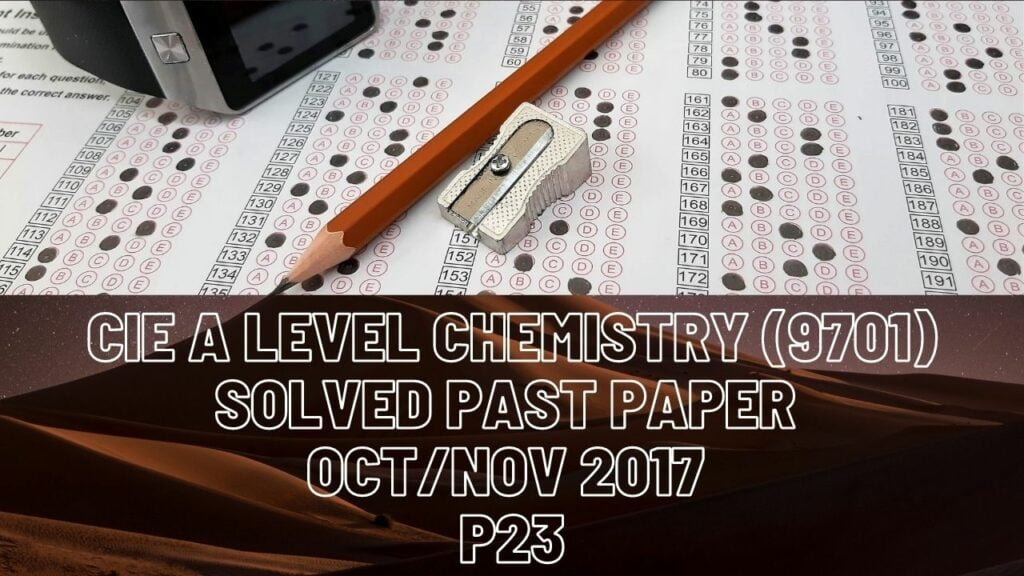A Level Chemistry### CIE A Level Chemistry Solved Past Paper Oct/Nov 2017 P23

Q1 a(i) The energy required to break one mole of bonds in gaseous form

(ii) x = (944+(3*436)+92)/6 = 391

b(i)  Diagram explained in video

(ii) Rate of production increases, as kinetic energy of reactant particles increases they collide more often. A greater proportion of molecules has energy greater than activation energy.

(iii) Yield decreases. The backward reaction is endothermic, equilibrium shifts to the left-hand side to oppose the change by decreasing temperature as energy is absorbed.

d(iii) Smaller container means greater pressure on reactant particles. High pressure favors the side with fewer molecules in equilibrium. This is the product side in this reaction, therefore the yield of ammonia increases. Kp remains unaffected because it is only affected by temperature.

Q2a(i) Energy required to remove an electron increases, because across the period number of protons in nucleus of atoms increases. This increases nuclear charge and nuclear attraction for outer shell electrons. Shielding effect remains fairly constant as number of shells remains constant.

(ii) First element has least shielding and hence greatest ionization energy. Second element has least nuclear charge and so the least ionization energy value.

(iii) Electron removed from Aluminiun is an electron removed from p orbital (opposed to electron removed from s orbital in magnesium). P orbital has experiences shielding and less nuclear attraction.

Electron removed from sulfur is from a filled 3p orbital (opposed to an electron removed from phosphorus from orbital containing a single electron). Electron pair repulsion due to same charge of two electrons decreases energy required to remove electron.

b(i) L-high melting point indicates giant ionic lattice. Mg(OH)2 has insoluble ppt in water as Na(OH) is soluble.

(ii) M-SiCl4 is only period 3 chloride that is hydrolysed in water and produces white solid.

Q3 a)  1-aqueous sodium hydroxide, substitution

2-ethanolic sodium hydroxide, elimination

3-ethanolic sodium cyanide, substitution

4-dilute sulfuric acid, hydrolysis

5-acidified chromate ions, oxidation

6-acidified chromate ions, oxidation

b)  Diagram explained in video

c(i) Isomers that have the same molecular formula and have different structural formula.

Position, functional group, or chain.

(ii) Sn1. This mechanism produces a tertiary carbocation that is more stable due to having 3 alkyl groups that have a greater positive inductive effect.

d(i) Stereoisomers have the same structural and molecular formula with different layout of atoms in space.

(ii) One of the two carbon atoms involved in C=C double bond is bonded to two hydrogen atoms. This means geometric isomerism is not expressed. The compound has no carbon atom that is single bonded to three different groups of atoms. This means the compound does not exhibit optical isomerism either.

(iii) X= 2-chlorobutane

Y= 1-chlorobutane

(iv) Optical isomerism

(v) Diagram explained in video